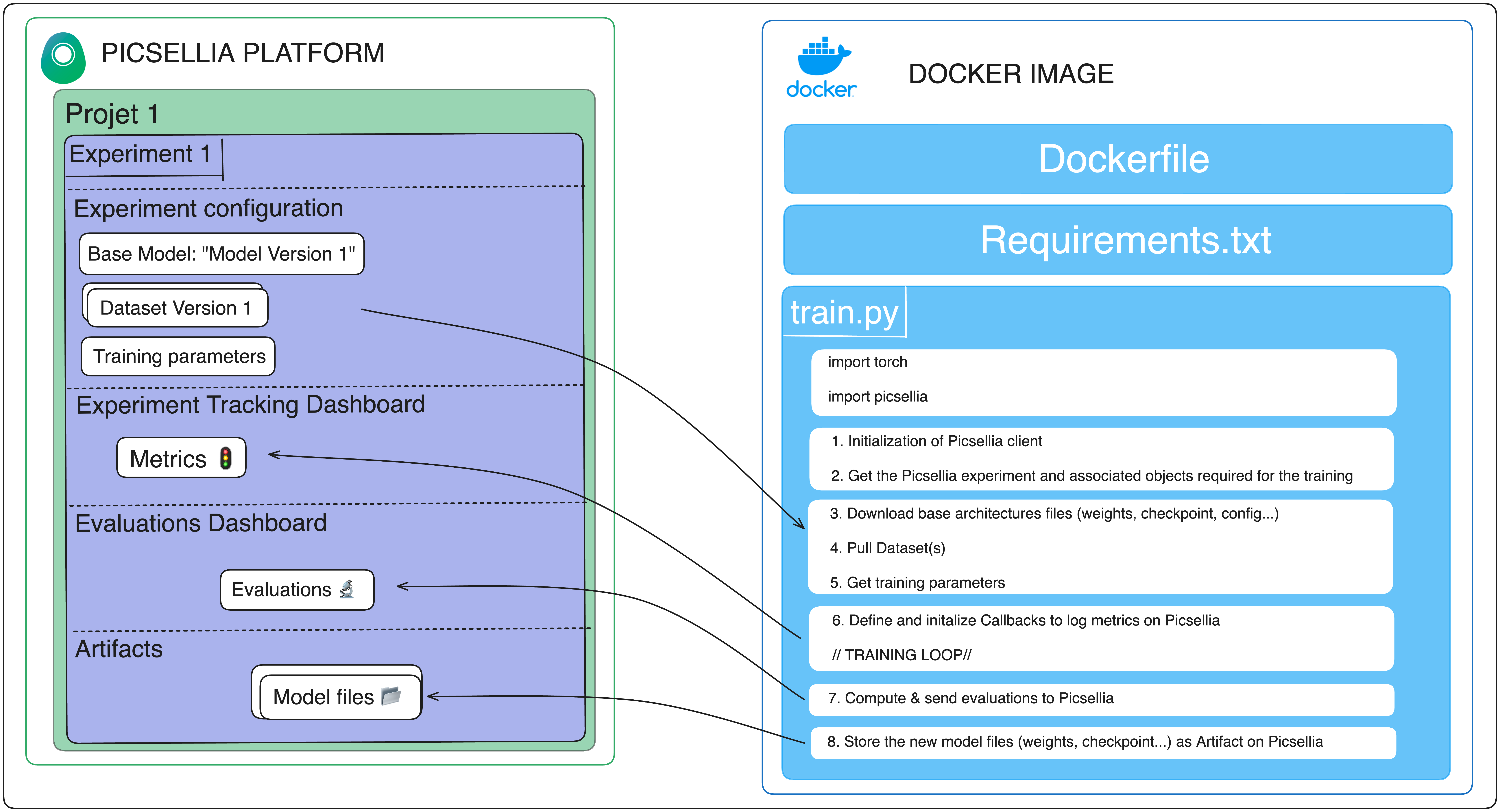# 1. Overview

This guide will focus on the development of a `main.py` script. At a high level, its structure will look like this:# 1. Prerequisite

To successfully follow this guide, you need to:

• Import at least one `Dataset` into Picsellia.
• Create a `Project` and attach a `Dataset` to it.
• Create an experiment with the attached `Dataset`.
• Have a `Model` and `ModelVersion`. If you don't have any, follow this tutorial to create them.

# 2. Starting point

Let's consider a folder containing three scripts: `model.py`, `utils.py`, and `train.py`.

For the purpose of this guide, we will focus on the `train.py` file and we will put all the code / functions inside. However, to enforce clean code conventions, it is recommended to create a dedicated file for Picsellia utilities.

Let's say we want to integrate Picsellia into an EfficientNet fine-tuning script, similar to this one from Keras, your `train.py` file should look like this:

``````from tensorflow.keras.applications import EfficientNetB0
from tensorflow.keras.models import Sequential
from tensorflow.keras import layers
import tensorflow as tf
import tensorflow_datasets as tfds

IMG_SIZE = 224

try:
tpu = tf.distribute.cluster_resolver.TPUClusterResolver.connect()
print("Device:", tpu.master())
strategy = tf.distribute.TPUStrategy(tpu)
except ValueError:
print("Not connected to a TPU runtime. Using CPU/GPU strategy")
strategy = tf.distribute.MirroredStrategy()

batch_size = 64

dataset_name = "stanford_dogs"
dataset_name, split=["train", "test"], with_info=True, as_supervised=True
)

NUM_CLASSES = ds_info.features["label"].num_classes

size = (IMG_SIZE, IMG_SIZE)
ds_train = ds_train.map(lambda image, label: (tf.image.resize(image, size), label))
ds_test = ds_test.map(lambda image, label: (tf.image.resize(image, size), label))

def format_label(label):
string_label = label_info.int2str(label)
return string_label.split("-")

label_info = ds_info.features["label"]

img_augmentation = Sequential(
[
layers.RandomRotation(factor=0.15),
layers.RandomTranslation(height_factor=0.1, width_factor=0.1),
layers.RandomFlip(),
layers.RandomContrast(factor=0.1),
],
name="img_augmentation",
)

# One-hot / categorical encoding
def input_preprocess(image, label):
label = tf.one_hot(label, NUM_CLASSES)
return image, label

ds_train = ds_train.map(
input_preprocess, num_parallel_calls=tf.data.AUTOTUNE
)
ds_train = ds_train.batch(batch_size=batch_size, drop_remainder=True)
ds_train = ds_train.prefetch(tf.data.AUTOTUNE)

ds_test = ds_test.map(input_preprocess)
ds_test = ds_test.batch(batch_size=batch_size, drop_remainder=True)

def build_model(num_classes):
inputs = layers.Input(shape=(IMG_SIZE, IMG_SIZE, 3))
x = img_augmentation(inputs)
model = EfficientNetB0(include_top=False, input_tensor=x, weights="imagenet")

# Freeze the pretrained weights
model.trainable = False

# Rebuild top
x = layers.GlobalAveragePooling2D(name="avg_pool")(model.output)
x = layers.BatchNormalization()(x)

top_dropout_rate = 0.2
x = layers.Dropout(top_dropout_rate, name="top_dropout")(x)
outputs = layers.Dense(NUM_CLASSES, activation="softmax", name="pred")(x)

# Compile
model = tf.keras.Model(inputs, outputs, name="EfficientNet")
model.compile(
optimizer=optimizer, loss="categorical_crossentropy", metrics=["accuracy"]
)
return model

with strategy.scope():
model = build_model(num_classes=NUM_CLASSES)

epochs = 25
hist = model.fit(ds_train, epochs=epochs, validation_data=ds_test, verbose=2)

def unfreeze_model(model):
# We unfreeze the top 20 layers while leaving BatchNorm layers frozen
for layer in model.layers[-20:]:
if not isinstance(layer, layers.BatchNormalization):
layer.trainable = True